# Gravitational Potential Energy | Definition, Derivation, Formula, Units – Gravitation

Gravitational Potential Definition Physics:
Gravitational potential at any point in gravitational field is equal to the work done per unit mass in bringing a very light body from infinity to that point. It is denoted by Vg.

We are giving a detailed and clear sheet on all Physics Notes that are very useful to understand the Basic Physics Concepts.

## Gravitational Potential Energy | Definition, Derivations, Formulas, Units – Gravitation

Gravitational Potential Formula:

PE = mgΔh

Where,
PEG = potential energy due to gravity.
g = acceleration due to gravity.
m = mass.
Δh = distance above a surface.

Gravitational Potential Derivation:

Gravitational potential, Vg = $$\frac{W}{m}=-\frac{G M}{r}$$

Gravitational Potential Units:
Its SI unit is J/kg and it is a scalar quantity.

Gravitational Potential Dimensional Formula:
Its dimensional formula is [L² T-2].
Since, work W is obtained, i.e. it is negative, the gravitational potential is always negative.

Gravitational Potential Energy Definition:
Gravitational potential energy of any object at any point in gravitational field is equal to the work done in bringing it from infinity to that point. It is denoted by U.

Gravitational Potential Energy Derivation:

Gravitational potential energy, U = $$-\frac{G M m}{r}$$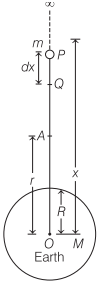The negative sign shows that the gravitational potential energy decreases with increase in distance.
Gravitational potential energy at height h from surface of earth

Uh = $$-\frac{G M m}{R+h}=\frac{m g R}{1+\frac{h}{R}}$$

Gravitational Potential Energy of a Two Particle System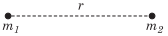The gravitational potential energy of two particles of masses m1 and m2 separated by a distance r is given by

U = $$-\frac{G m_{1} m_{2}}{r}$$

Gravitational Potential Energy for a System of More than Two Particles
The gravitational potential energy for a system of particles (say m1, m2, m3 and m4) is given by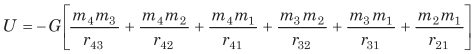Thus, for a n particle system there are $$\frac{n(n-1)}{2}$$ pairs and the potential energy is calculated for each pair and added to get the total potential energy of the system.

### Gravitational Potential for Different Bodies

1. Gravitational Potential due to a Point Mass:
Suppose a point mass M is situated at a point O, the gravitational potential due to this mass at point P is given by

V = $$-\frac{G M}{r}$$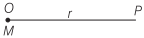2. Gravitational Potential due to Ring: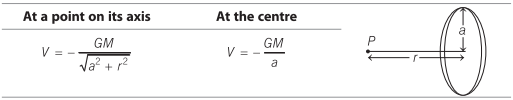3. Gravitational Potential due to Spherical Shell: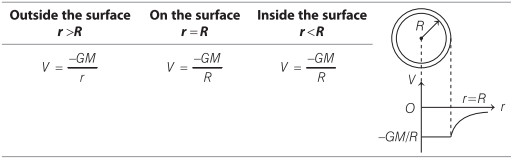4. Gravitational Potential due to Solid Sphere:Gravitation:
Have you ever thought, when we throw a ball above the ground level, why it returns back to the ground. It’s because of gravity. When a ball is thrown above the ground in the opposite direction, a gravitational force acts on it which pulls it downwards and makes it fall. This phenomena is called gravitation.

Learn relation between gravitational field and potential field, Kepler’s law of planetary, weightlessness of objects in absence of gravitation, etc.